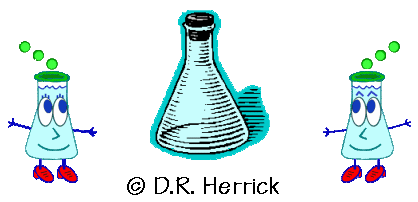Dr. Herrick's CH221 Pressure Quiz 5Thermochemistry Copyright D. Herrick20 Questions from past exams. Practice for speed.  Aim for 2 minutes per problem.
 1 Which of the following are not state functions? I-heat,  II-work,  III-energy,  IV-enthalpy
 A)  I B)  I,  II C)  III,  IV D)  none E)  all
 2 The first law says the energy of a system is conserved if the system is
 A) at constant temperature B) at constant volume C) at constant pressure D) isolated from the surroundings E) thermally insulated from the surroundings
 3 The convention is that work done by the system is
 A)  positive B)  zero C)  negative
 4 The work (w) for a process that has  DE = 56 J and q = - 40 J is
 A) 16 J B) -96 J C) zero D) -16 J E) 96 J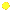5 Given the following specific heat capacities of metals, which metal will reach the highest temperature if the same amount of heat is added to 25 g samples of each of the metals initially at 20.0ºC?
 A) aluminum (MW = 26.98) 0.900 J/g-ºC B) calcium (MW =40.08) 0.653 J/g-ºC C) copper (MW = 63.55) 0.385 J/g-ºC D) gold (MW = 197.0) 0.129 J/g-ºC E) nickel (MW = 58.69) 0.444 J/g-ºC
 6 Repeat Question 5 for 1 mole samples of each metal.
 A) aluminum D) gold B) calcium E) nickel C) copper
 7 The first law says the energy change for a system is equal to
 A) the heat absorbed by the system plus the work done by the system B) the heat absorbed by the system plus the work done on the system C) the heat emitted from the system plus the work done by the system D) the heat emitted from the system plus the work done on the system E) none of the above8 If a chemical reaction at constant pressure gives off heat to the surroundings the enthalpy change of the reaction is
 A)  positive B)  zero C)  negative
 9 The reaction in Question 8 is called
 A) endoergic B) exoergic C) endothermic D) exothermic E) hypothermic
 10 Calculate the enthalpy change of 450 g of water as it cools from 90.0ºC to 30.0ºC.  The heat capacity of water is 4.184 J/g-ºC.
 A) 27 kJ D) -6 kJ B) 113 kJ E) -113 kJ C) 6 kJ F) -27 kJ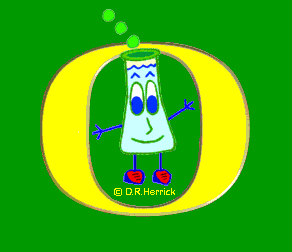11 Given these reactions2 P(s) + 3 Cl2(g)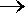2 PCl3(l) ,   DHº = -636 kJ PCl5(s)PCl3(l) +  Cl2(g) ,     DHº = 137 kJ the standard heat of formation of solid PCl 5 is
 A) -499 kJ B) -455 kJ C) -568 kJ D) -181 kJ E) - 44 kJ
 12 Given these gas reactions2 HClH2 + Cl2 ,   DHº = 185 kJ 2 H2 + O22 H2O ,   DHº = -484 kJ calculate DHº for the following reaction: H2O + Cl22 HCl + 0.5 O2
 A)  -299 kJ B)  669 kJ C)  427 kJ D)  57 kJ E)  none of the above
 13 Given these atom reactions NaNa+ + e-  ,   DHº = 502 kJ ClCl+ + e-  ,     DHº = 1530 kJ Na  + e-Na-,     DHº = -53 kJ Cl  + e-Cl-,       DHº = -349 kJ calculate DHº for the following ion reaction Na+ + Cl-Na- + Cl+
 A) 1324 kJ B) 1430 kJ C) 2434 kJ D) 732 kJ E) 626 kJ
 14 When an endothermic reaction is carried out in a constant pressure calorimeter the temperature of the surrounding water bath is expected to
 A)  increase B)  decrease A)  remain unchanged
15. Use the standard heats of formation
 Al2O3(s) -1676 kJ HF(l) -300 kJ H2O(l) -286 kJ NaAlF4(s) -2017 kJ NaClO3(s) -361 kJ Na2CO3(s) -1131 kJ Na2O(s) -417 kJ SnCl2(s) -350 kJ

to determine DHº  for the reaction

0.5 Na2O(s) + 0.5 Al2O3(s) + 4 HF(l)NaAlF4(s) + 2 H2O(l)

 A) -4696 kJ D) -4836 kJ B) 1217 kJ E) 90 kJ C) -156 kJ F) -342 kJ16 Given the reactionA + 2 B3 C,    DHº =  24 kJ find DHº  for the reaction 3 CA + 2 B
 A) -12 kJ B) -24 kJ C) -36 kJ D) -48 kJ E) -64 kJ
 17 Calculate the heat of formation of NO from the following heats of reaction:4NH3 + 5 O24 NO + 6 H2O ,   DHº = -1170 kJ 4NH3 + 3 O22 N2 + 6 H2O ,    DHº = -1530 kJ
 A) -180 kJ D) 90 kJ B) 180 kJ E) -360 kJ C) -90 kJ F) 360 kJ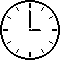18 What's the final temperature after a 20 kg bar of iron at 25ºC absorbs 540 kJ of heat?  The specific heat capacity of iron is 0.450 J/g-ºC.
 A) 60ºC D) 70ºC B) 75ºC E) 80ºC C) 85ºC F) 65ºC
 19 Given the reactionA + 2 B3 C,    DHº =  24 kJ find DHº  for the production of 0.25 mole of C.
 A) 2 kJ D) 96 kJ B) 4 kJ E) 18 kJ C) 6 kJ F) 72 kJ
 20 Given the reactionsA + 2 B3 C,    DHº =  24 kJ 2 CE,              DHº =  12 kJ what is DHº  for the production of 24 moles of E from A and  B.
 A) 504 kJ D) 108 kJ B) 288 kJ E) 672  kJ C) 84 kJ F) 192 kJ